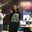Trusted answers to developer questions
Trusted Answers to Developer Questions

Related Tags

theoretical cs

# What is the prenex normal form?Ayesha Kanwal

Grokking Modern System Design Interview for Engineers & Managers

Ace your System Design Interview and take your career to the next level. Learn to handle the design of applications like Netflix, Quora, Facebook, Uber, and many more in a 45-min interview. Learn the RESHADED framework for architecting web-scale applications by determining requirements, constraints, and assumptions before diving into a step-by-step design process.

## Overview

The prenex normal form is a method to deal with formulas so that the quantifiers are moved in front of the expression.

### Prenex normal form

The prenex normal form is written as:

In this form $Q_i$ is $\forall$ or $\exists$ where $i = 0, 1,2...n$ and $F$ is the quantifier-free formula. $Q_1x_1 \ Q_2x_2...Q_nx_n$ is called the prefix, whereas $F$ is called the matrix.

A formula with no quantifiers is called a trivial case of the prenex normal form.

### Steps to convert into PNF

We'll follow the steps below to convert any expression into PNF:

1. We eliminate all the occurrences of $\rightarrow$ and $↔$ from the formula.
2. We move all the negations inwards to appear only as a part of the literal.
3. Standardize the variables apart if it is necessary.
4. PNF is obtained by moving the quantifiers to the front of the formula.

#### Step 1

To remove the conditional $\rightarrow$ (if $A$ then $B$) and bi-conditional $↔$ ($A$ if and only if $B$), we use the following logical equivalences:

• $A \rightarrow B \equiv \neg A \vee B$
• $A ↔ B \equiv (\neg A \vee B) \wedge (A \vee \neg B)$
• $A ↔ B \equiv (A \wedge B) \vee (\neg A \wedge \neg B)$

#### Step 2

We'll now try to move all the negations close to the literals instead of the negations occurring as a whole. We convert the $\forall$ symbol to the $\exists$ symbol and vice versa in this step when we shift the $\neg$ symbol. To accomplish step 2, we use the following logical equivalences:

• $\neg \neg A \equiv A$
• $\neg \forall xA(x) \equiv \exists x \neg A(x)$
• $\neg \exists xA(x) \equiv \forall x \neg A(x)$
• De Morgan's law

#### Step 3

Renaming of the variables is called the standardizing of the variables apart. To achieve step 3, we use the following theorem to rename the variables to make them distinct.

Suppose we get $A'$ from $A$ by making some replacements in $A$ of the occurrences of $Q(y)F(y)$ by $Q(x)F(x)$. $Q$ can either be an existential or universal quantifier.

#### Step 4

In this step, we'll shift all the $\forall$ and $\exists$ to the beginning of the expression. To achieve step 4, we use the following basic logical equivalences:

• $A \vee \forall xF(x) \equiv \forall x(A \vee F(x))$ where $x$ does not occur in $A$.
• $A \vee \exists xF(x) \equiv \exists x(A \vee F(x))$ where $x$ does not occur in $A$.
• $A \wedge \forall xF(x) \equiv \forall x(A \wedge F(x))$ where $x$ does not occur in $A$.
• $A \wedge \exists xF(x) \equiv \exists x(A \wedge F(x))$ where $x$ does not occur in $A$.

### Example

Let's consider the following expression:

To convert it into PNF, we follow the steps mentioned above.

#### Step 1

We'll eliminate $\rightarrow$ and $↔$ from the expression.

#### Step 2

We'll move the negations inwards.

#### Step 3

We'll standardize the variables.

#### Step 4

We'll now move the quantifiers to the front, and this gives us:

#### Output

In conclusion,

This is the final PNF form of the expression.

RELATED TAGS

theoretical cs

CONTRIBUTORAyesha Kanwal